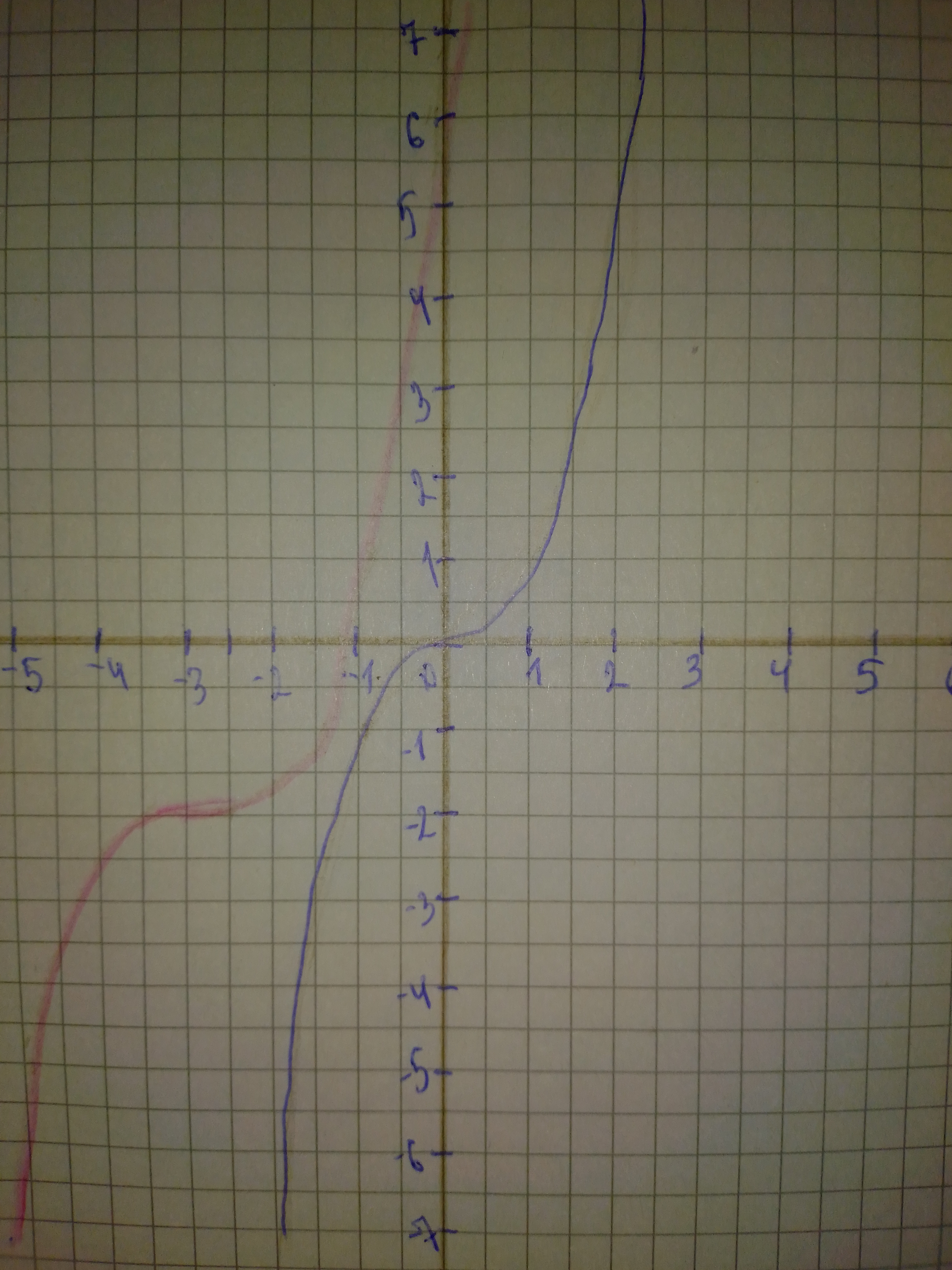# a) Identify the parameters a, b, h, and k in the polynomial y=frac{1}{3}(x+3)^{3}-2 Describe how each parameter transforms the base function y=x^{3}. b) State the domain and range of the transformed function. c) Sketch graphs of the base function and the transformed function on the same set of axes.Question
Polynomial graphsa) Identify the parameters a, b, h, and k in the polynomial $$\displaystyle{y}={\frac{{{1}}}{{{3}}}}{\left({x}+{3}\right)}^{{{3}}}-{2}$$ Describe how each parameter transforms the base function $$\displaystyle{y}={x}^{{{3}}}$$.
b) State the domain and range of the transformed function.
c) Sketch graphs of the base function and the transformed function on the same set of axes.2021-02-26
Step 1
Work as shown below, follow the steps:
a) Use the general transformation equation: $$\displaystyle{y}={a}{f{{\left({b}{\left({x}-{h}\right)}\right)}}}+{k}$$
We are given the transformed function: $$\displaystyle{y}={\frac{{{1}}}{{{3}}}}{\left({x}+{3}\right)}^{{{3}}}-{2}$$
By finding the values of the parameters a,b,c,d we see that the graph of the parent function $$\displaystyle{y}={x}^{{{3}}}$$ will undergo the following transformations:
-No horizontal stretch, because $$\displaystyle{b}={1}$$
-A vertical stretch by a factor of $$\displaystyle{\frac{{{1}}}{{{3}}}}$$ because $$\displaystyle{a}={\frac{{{1}}}{{{3}}}}$$
-A horizontal translation 3 units left because $$\displaystyle{h}=-{3}{<}{0}$$</span>
-A vertical translation 2 units down because $$\displaystyle{k}=-{2}{<}{0}$$</span>
Step 2
For the domain:
The end behavior for this function is Down on the left and Up on the right, so this function can get all values in the set of real numbers (vertical axis) so:
The range is: $$\displaystyle{R}={\left\lbrace{y}{\mid}{y}\in{R}\right\rbrace}$$
Step 3
For the graph of the given transformed function start from the graph of the parent function $$\displaystyle{y}={x}^{{{3}}}$$
Apply the transformations from part a) to the graph of $$\displaystyle{y}={x}^{{{3}}}$$ to obtain the graph of the transformed function $$\displaystyle{y}={\frac{{{1}}}{{{3}}}}{\left({x}+{3}\right)}^{{{3}}}-{2}$$
See in the following picture:### Relevant Questionsa) Identify the parameters a, k, d, and c in the polynomial function $$\displaystyle{y}={\frac{{{1}}}{{{3}}}}{\left[-{2}{\left({x}+{3}\right)}\right]}^{{{4}}}-{1}$$. Describe how each parameter transforms the base function $$\displaystyle{y}={x}^{{{4}}}$$. b) State the domain and range, the vertex, and the equation of the axis of symmetry of the transformed function. c) Describe two possible orders in which the transformations can be applied to the graph of $$\displaystyle{y}={x}^{{{4}}}$$ to produce the graph of $$\displaystyle{y}={\frac{{{1}}}{{{3}}}}{\left[-{2}{\left({x}+{3}\right)}\right]}^{{{4}}}-{1}$$. d) Sketch graphs of the base function and the transformed function on the same set of axes.Rational functions can have any polynomial in the numerator and denominator. Analyse the key features of each function and sketch its graph. Describe the common features of the graphs. $$\displaystyle{a}{)}{f{{\left({x}\right)}}}={\frac{{{x}}}{{{x}^{{{2}}}-{1}}}}\ {b}{)}{g{{\left({x}\right)}}}={\frac{{{x}-{2}}}{{{x}^{{{2}}}+{3}{x}+{2}}}}\ {c}{)}{h}{\left({x}\right)}={\frac{{{x}+{5}}}{{{x}^{{{2}}}-{x}-{12}}}}$$(a) find the Maclaurin polynomial $$\displaystyle{P}_{{{3}}}{\left({x}\right)}$$ for f(x), (b) complete the following $$\displaystyle{x}:-{0.75},-{0.50},-{0.25},{0},{0.25},{0.50},{0.75}{f}{\quad\text{or}\quad}{f{{\left({x}\right)}}}$$ and $$\displaystyle{P}_{{{3}}}{\left({x}\right)}$$, and (c) sketch the graphs of f(x) and $$\displaystyle{P}_{{{3}}}{\left({x}\right)}$$ on the same set of coordinate axes. $$\displaystyle{f{{\left({x}\right)}}}={\arcsin{{x}}}$$(a) find the Maclaurin polynomial $$\displaystyle{P}_{{{3}}}{\left({x}\right)}$$ for PSKf(x), (b) complete the following PSKx: -0.75, -0.50, -0.25, 0, 0.25, 0.50, 0.75 for f(x) and P_{3}(x) and (c) sketch the graphs of f(x) and $$\displaystyle{P}_{{{3}}}{\left({x}\right)}$$ on the same set of coordinate axes. $$\displaystyle{f{{\left({x}\right)}}}={\arctan{{x}}}$$Find the quadratic polynomial $$\displaystyle{g{{\left({x}\right)}}}-{a}{x}^{{{2}}}\ +\ {b}{x}\ +\ {c}\ \text{which best fits the function}\ {f{{\left({x}\right)}}}={e}^{{{x}}}\ \text{at}\ {x}={0},\ \text{in the sense that}\ {g{{\left({0}\right)}}}={f{{\left({0}\right)}}},\ \text{and}\ {g}'{\left({0}\right)}={f}'{\left({0}\right)},\ \text{and}\ {g}{''}{\left({0}\right)}={f}{''}{\left({0}\right)}.$$ Using a computer or calculator, sketch graphs of f and g on the same axes. What do you notice?Graph the polynomial in the given viewing rectangle. Find the coordinates of all local extrema. State each answer rounded to two decimal places. State the domain and range. $$\displaystyle{y}={x}^{{{3}}}-{3}{x}^{{{2}}},{\left[-{2},{5}\right]}{b}{y}{\left[-{10},{10}\right]}$$Using calculus, it can be shown that the arctangent function can be approximated by the polynomial
$$\displaystyle{\arctan{\ }}{x}\ \approx\ {x}\ -\ {\frac{{{x}^{{{3}}}}}{{{3}}}}\ +\ {\frac{{{x}^{{{5}}}}}{{{5}}}}\ -\ {\frac{{{x}^{{{7}}}}}{{{7}}}}$$
a) Use a graphing utility to graph the arctangent function and its polynomial approximation in the same viewing window. How do the graphs compare?
b) Study the pattern in the polynomial approximation of the arctangent function and predict the next term. Then repeat part (a). How does the accuracy of the approximation change when an additional term is added?Using calculus, it can be shown that the secant function can be approximated by the polynomial $$\displaystyle{\sec{{x}}}\approx{1}+{\frac{{{x}^{{{2}}}}}{{{2}!}}}+{\frac{{{5}{x}^{{{4}}}}}{{{4}!}}}$$ where x is in radians. Use a graphing utility to graph the secant function and its polynomial approximation in the same viewing window. How do the graphs compare?a) Sketch graphs of $$\displaystyle{y}={\sin{\ }}{x}$$ and $$\displaystyle{y}={\cos{\ }}{x}.$$Investigate the change in the graph of a sinusoidal function of the form $$\displaystyle{\quad\text{and}\quad}={\sin{{x}}}{\quad\text{or}\quad}{\quad\text{and}\quad}={\cos{{x}}}$$ when multiplied by a polynomial function. Use a graphing calculator to sketch the graphs of and $$\displaystyle={2}{x},{\quad\text{and}\quad}=-{2}{x},{\quad\text{and}\quad}={2}{x}{\cos{{x}}}$$ on the same coordinate plane, on the interval $$\displaystyle{\left[-{20},{20}\right]}.$$